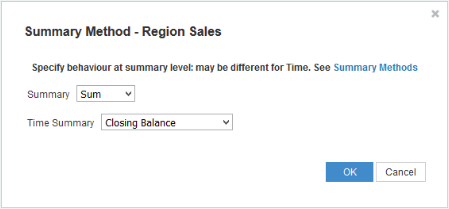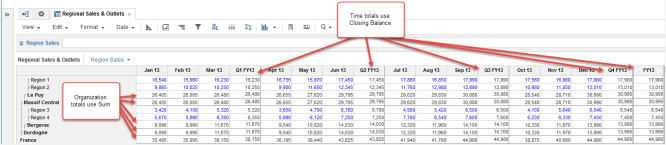1. Modeling
2. Build Models
3. Summary Method

Use Summary Method to define how line item totals are calculated.

Learn how to specify a summary method for your line items, what summary methods are available for different line item formats, what these options mean, and how to set a different time Summary Method.

## Select a Summary Method

1. Open Blueprint.
2. In the Summary column for a line item, click the ellipsis: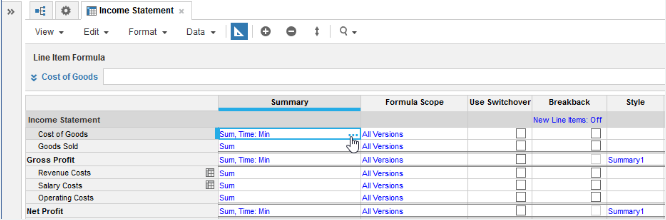The Summary Method dialog displays: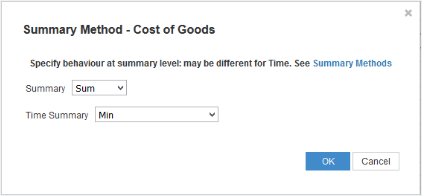1. Select a summary method from the Summary list. If the module dimensions include both a list and Time, you can set the summary method on each of them independently.

## Available Summary Methods by line item format

Each line item format is compatible with a range of summary methods for its totals. This table shows which summary methods work with which line item formats:

 Summary Method Number Date List Time Period Text Boolean Sum Yes None Yes Yes Yes Yes Yes Yes Formula Yes Yes Yes Yes Yes Yes Average Yes Ratio Yes Min Yes Yes Yes Max Yes Yes Yes Opening Balance (Time Summary only) Yes Closing Balance (Time Summary only) Yes Any Yes All Yes First non-blank Yes Yes Yes Yes Last non-blank Yes Yes Yes Yes

## Sum

Adds values over time and other hierarchical aggregations.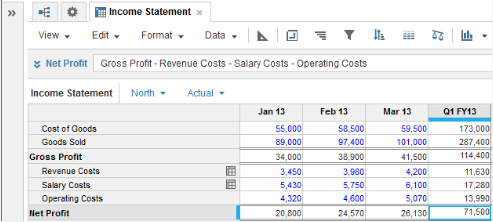Here, Sum is set as the main summary method for each line item. The time summary is set as Same as main summary, so quarterly values are sums of monthly values.

## None

Displays totals as blank or zero.

## Formula

Aggregates first, then applies the formula to the totals.

It makes no sense to add some items, such as headcount, balances, prices, ratios, or percentages. For example, imagine the price of an item for Jan, Feb and Mar is 12, 13 and 10 respectively. Sum would return 12+13+10 = 35, which is not meaningful.

In such cases, set the summary item as Formula if the line item contains a formula. If it contains no formula, set the summary as None, which returns a blank. When you set the summary method as Formula, it applies the formula to the aggregated amounts.

For example, we have two line items named Cost of Goods and Units Purchased. A third line item, Unit Cost Price, equals Cost of Goods divided by Units Purchased. In this scenario, it makes no sense to add up the prices to calculate the quarterly total. It makes much more sense to set the summary as Formula. The Formula summary method uses the same formula as the Unit Cost Price line item, so divides the quarterly sales by units sold. This is more logical and useful than a total of the prices for January, February, and March.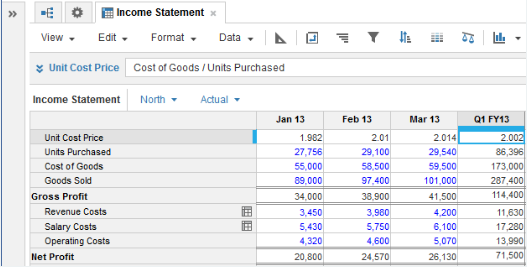## Average

Calculates the mean average for values over time and other hierarchical aggregations.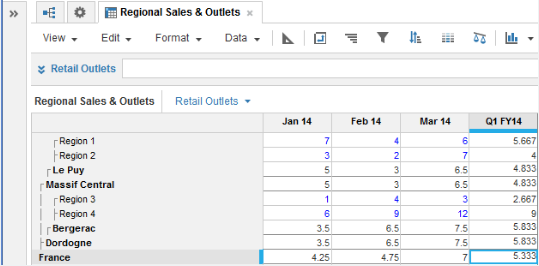## Ratio

Uses a ratio of two specified line items for the totals.

When setting up a Ratio summary method, you must specify the line items used in the ratio.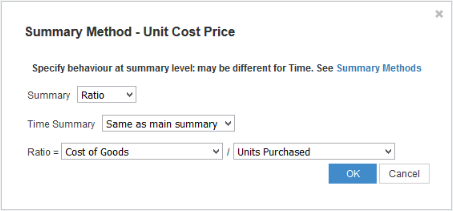In this example, Ratio is used to work out the cost price of the goods purchased.

## Min

Uses the smallest value for the totals.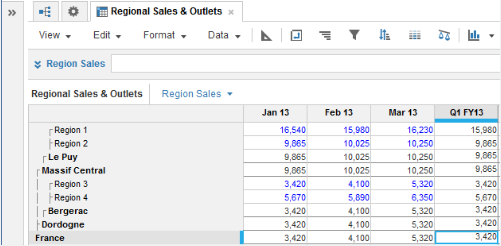If you use Min as the summary method, it returns Infinity for any parent items that have no child items or descendants: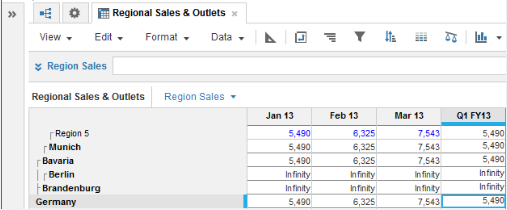Here, the German state of Brandenburg and city of Berlin are parent items without any child items or descendants in the Regions list.

## Max

Uses the largest value for the totals.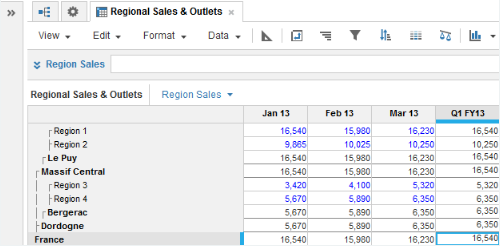If you use Max as the summary method, it returns -Infinity for any parent items that have no child items or descendants: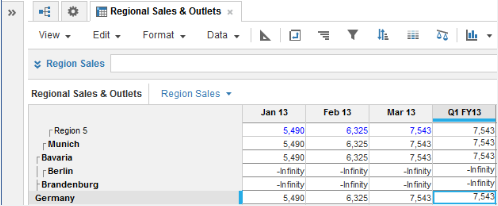Here, the German state of Brandenburg and city of Berlin are parent items without any child items or descendants in the Regions list.

## Opening Balance

Uses the value from the first summarized time period for totals. Only compatible with number-formatted line items with a Time dimension.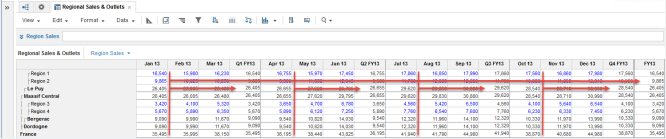## Closing Balance

Uses the value from the last summarized time period for totals. Only compatible with number-formatted line items with a Time dimension.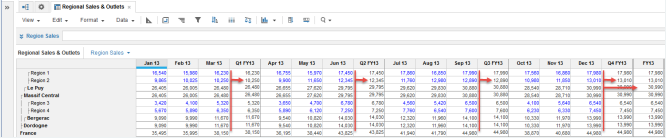## All

Returns a value of true for totals if all summarized values are also true. Only compatible with Boolean-formatted line items.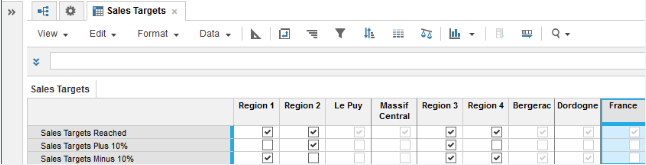## Any

Returns a value of true for totals if any of the summarized values are true. Only compatible with Boolean-formatted line items.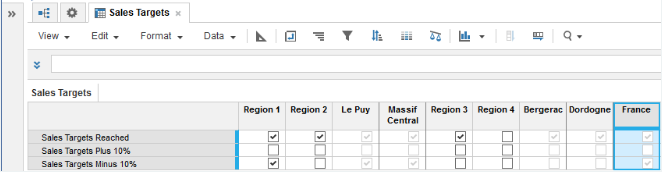## First non-blank

Returns the first item that contains data in a list for the totals. The first item is the one that displays at the top when you open a list in General Lists. This may differ from the order in your module. You can change the order of lists with the Order List action.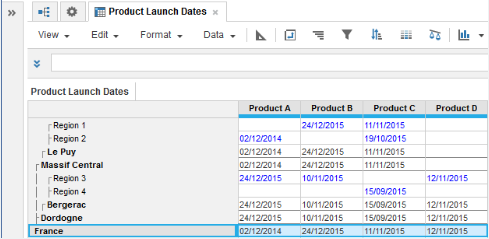## Last non-blank

Returns the last item that contains data in a list for the totals. The last item is the one that displays at the bottom when you open a list in General Lists. This may differ from the order in your module. You can change the order of lists with the Order List action.

## Select a different Summary Method for Time

In this example, a different summary method is used for Organization and Time.

Sum is set for Summary, which means that the Organization totals use addition. Closing Balance is set for the Time Summary, which means that the Time totals use the value from the last time period.